# Concept 9 – Proportions and Percents

[expand title=”How can I make it bigger?”]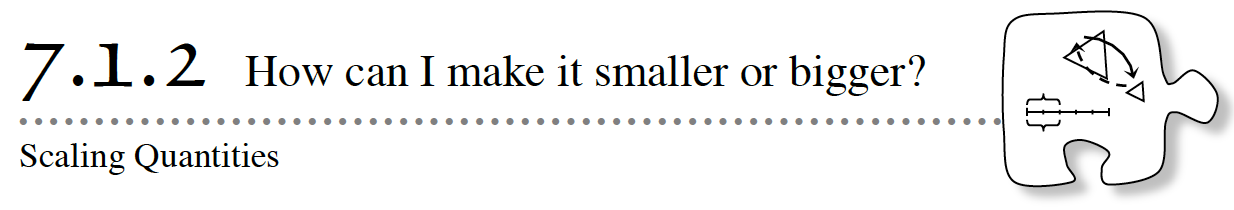7-16. Dana is training for a bicycle race.  He can ride his bike 25 miles per hour.

One day, when he had been riding forof an hour, he had to stop and fix a flat tire.  How many miles had he ridden when he stopped?  The diagram below may be useful.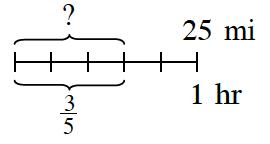7-17. Matt thought about problem 7-16 and drew the diagram at right.  Look at Matt’s drawing and decide how he is thinking about this problem.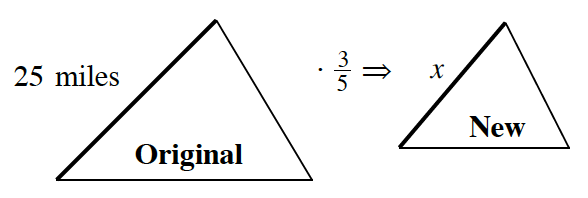a. Write an equation that uses the scale factor to find  x.

b. What connection is Matt making between finding a distance using the rate and time (as you did in problem 7-16) and using a scale factor with similar figures?  How are the situations alike and how are they different?

7-18. In the two previous problems,is used in two ways: first, as time in the rate problem ashrs, and second, as the scale factor in the similar triangle problem used to find three fifths of 25 miles.  Both of these situations resulted in an equivalent calculation: 25 ·= 15.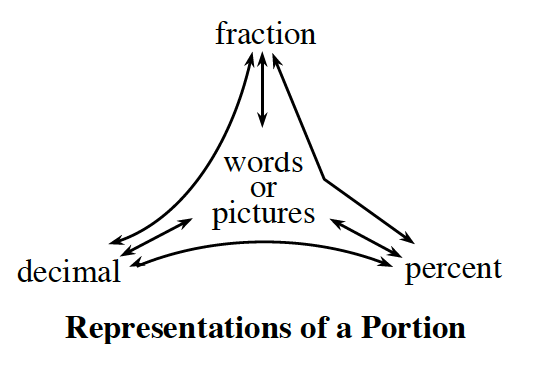a. Using the portions web shown at right, work with your team to find two other ways to write the equation 25 ·= 15.  For example, one way might be 25 ·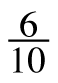= 15.

b.  If you did not already find it, what percent would be equivalent to?  Use this percent to write a statement in words and symbols that is equivalent to 25 ·= 15.

c. Use the idea of scaling to find the following values.  Write an expression using either a fraction or a decimal, and then find the result.

1. 90% of 25 miles
2. 8% of \$75
3. 25% of 144

7-19. Josea went out to dinner at an Indian restaurant.  The total bill was \$38.  She wanted to leave a 15% tip.

a. If you use the idea of scaling to find the tip amount, what would she need to multiply by?  As you talk about this with your team, consider:

• How could you represent this multiplier as a fraction?
• How could you represent it as a decimal?
• Does it make a difference which representation, fraction or decimal, you use to solve this problem?
• Which do you think will be easier?

b. How much should Josea leave for the tip?  Show your calculations.

c. If Josea changes her mind and wants to leave a 20% tip instead, how much will this be?

7-20. While shopping for a computer game, Isaiah found one that was on sale for 35% off.  He was wondering if he could use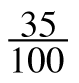as a multiplier to scale down the price to find out how much he would have to pay for the game.

a. If Isaiah usesas a scale factor (multiplier), will he find the price that he will pay for the game?  Why or why not?

b. There is scale factor (multiplier) other than 35% that can be used to find the sale price.  What is it?  Draw a diagram to show how this scale factor is related to 35%.  Label the parts of your diagram “discount” and “sale price” along with the relevant percents.

c. How much will Isaiah have to pay for the game if the original price is \$40?  Show your strategy.

Assignment: 7.1.2 Homework

[/expand]

[expand title=”Which multiplier should I use?”]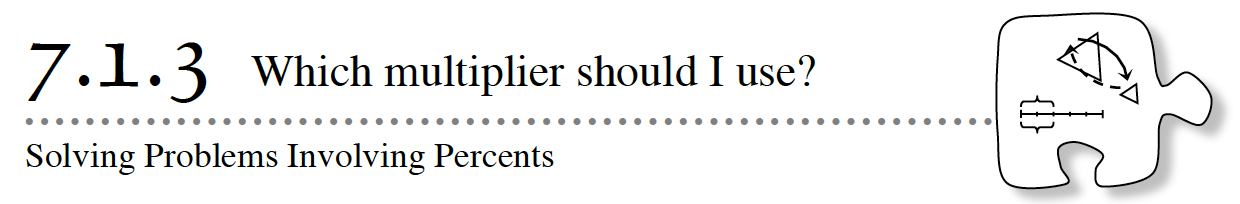7-27. Hugo and his family were shopping and purchased a new bed.  The bed was a great deal at 60% off of the original cost.  The bed originally cost \$245.

a. Draw a diagram for this situation.

b. If Hugo scales (multiplies) the original price of the bed by 60%, what will his result represent?

c. What should Hugo scale (multiply) the original price by to find the new price of the bed?

d. Work with your team to find the sale price of the bed in two different ways, that is, using two different multipliers (scale factors).  How do your answers from your two methods compare?

7-28. Hugo’s older sister, Sandra, had the same summer job for the past two years.  Last year, she worked the entire summer and was paid a salary of \$3,000.  This summer, she is going to get a 6% raise in pay.  To figure out how much she will make, Sandra drew the following diagram.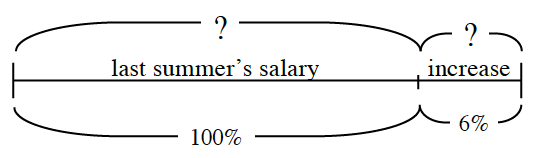a. Copy the diagram on your paper and fill in the missing information.

b. Since Sandra’s salary is increasing, does it make sense that her scale factor (multiplier) should be less than 1, equal to 1, or more than 1?  Why?

c. The diagram shows Sandra’s original salary and the amount of the increase.  What is the scale factor (multiplier) between her original salary and her new salary?  That is, what number could Sandra multiply the original salary by to get the new salary?

d. Show two ways that use different scale factors (multipliers) that Sandra can use to compute her new salary.

7-29. Miranna teaches gymnastics lessons at summer camp.  She is paid \$12.50 per hour.

a. If Miranna were offered a raise of 100% per hour, what would her new hourly rate be?  What percent of her original pay would she be paid?

b. Miranna is offered a raise of 75% of her hourly rate to teach a private lesson.  How much per hour would she be paid for the private lesson?
What percent of her original pay would she get?

c. What is the relationship between the percent raise that Miranna gets and her new pay as a percent of her original pay?  How is this related to the scale factor  (multiplier) between her original pay and her new pay?7-30. Liam was working on a problem and drew a diagram like the one below.  He then wrote the equation (\$72)(0.14) = x.  Does his equation agree with his diagram?

a. If you agree, then solve his equation for  x.

b. If you disagree, write and solve a new equation that will find   in his diagram.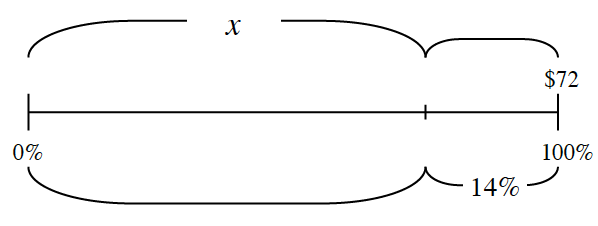Assignment: 7.1.3 Homework

[/expand]

[expand title=”Percent of What?”]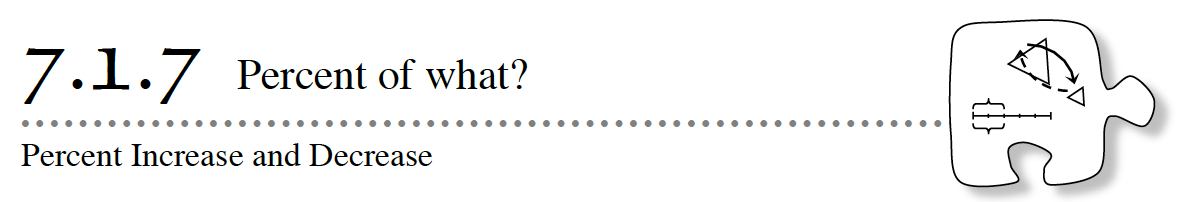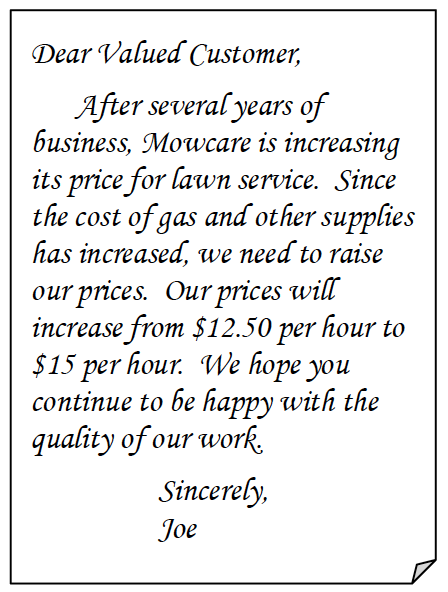7-76. WHAT IS THE PERCENT INCREASE?

Several years ago, Joe started a lawn-care business.  Due to rising costs, he needs to increase his prices.  He is concerned, however, because he has heard that if he raises his rates more than 33%, he might lose business.  At right is a letter he sent to his clients.

Joe began to wonder about how the increase in his fees could be expressed in terms of a percent.

a. Joe wants to determine the scale factor (multiplier) for the price increase and wrote the following equation:

12.50x = 15

Explain how this expression represents this situation.  What does   represent?

b. Solve the equation above to determine the scale factor for his change in fees.  Represent this multiplier (scale factor) as a fraction, as a decimal, and as a percent.

c. How could this situation be represented with a line diagram?  With your team, copy and complete the diagram below.  Then answer and do the following: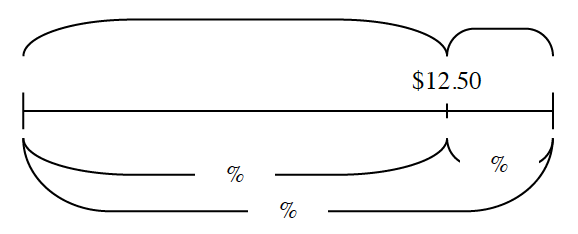• Which portion of the diagram corresponds to the original price?  Circle and label this part “original.”
• Which portion of the diagram corresponds to the change in price?  Circle and label this part “change.”

d. When Joe raised his rates, what was the percent increase?  That is another way of asking: What percent of the original price did the price change?  Should Joe expect to lose business?

7-77. Paige needs your help!  She wants to convince her grandmother to let her sign up for a rock-climbing class.  The class normally costs \$50, but the school is offering a special price of \$34.  Paige’s grandmother wants to know what percent of the cost of the class she would save.

a. Without calculating, estimate the percent of the discount.

b. With your team, determine the percent change in the price of the class.  Use the prompts below to help guide your team’s discussion.

• Draw a diagram or write an equation that represents the situation.
• What is the original (whole) value?  Indicate this on your diagram or in your equation.
• What percent is the change?  Find and indicate this on your diagram or in your equation.
• Does this situation represent an increase or decrease?

7-78. The percent change is a comparison of the amount of change to the original amount.  If a number increases from the original amount, it is called percent increase.  If the number decreases from the original, it is called a percent decrease.

a. What is the percent change from \$30 to \$33?  Is this a percent increase or decrease?  To answer this question:

• Draw a diagram to represent the problem.
• Determine if it is a percent increase or decrease.
• Calculate the percent change.

b. What is the percent change from \$33 to \$30?  Is this a percent increase or decrease?  To answer this question:

• Draw a diagram to represent the problem.
• Determine if it is a percent increase or decrease.
• Calculate the percent change.

c. If both parts (a) and (b) above have a change of \$3, why are the percent changes different? Explain.

7-79. To attract new customers, a shoe company called Shoe Fits will raise its prices 10% and then offer a 10%-off coupon.

a. Without doing any calculations, consider the following question: If a coupon is used, do you think the final price will be more than, less than, or the same as the original price?  Discuss this with your team and be ready to share your thinking with the class.

b. Akari wants to use a coupon to buy a pair of shoes that originally cost \$46.  What will the increased price be?  What will be the price after the 10%-off coupon is used?  Show all calculations.  Does this result confirm your answer to part (a)?

c. Akari had assumed that if the price increased 10% and then he received a 10% discount, it would return to the original price.  Explain why this did not happen.

Assignment: 7.1.7 Homework

[/expand]

[expand title=”How does it change over time?”]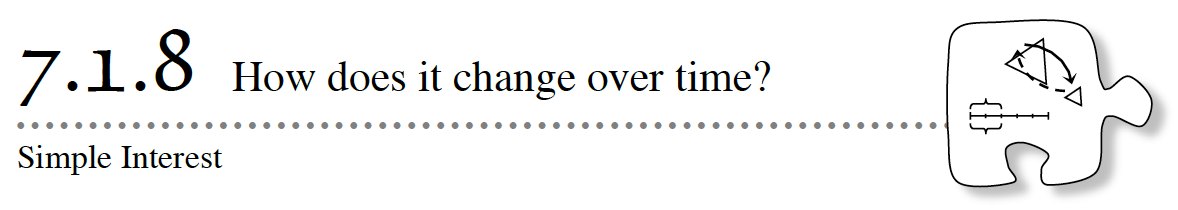7-86. RENTING THE HALL

The student council is planning a spring celebration.  Unfortunately, to rent a hall for the event, they will need to pay a deposit in advance of the event.  Since they have not started selling tickets yet, they asked the Parent-Teacher Association for a loan of \$825 for the hall rental.  The PTA agreed, but said it would charge 2% simple interest each week until the loan is paid back.

Ms. Becker, the student-council advisor, explained to the students that the amount of the loan (\$825) is called the principal amount.  Until the loan is paid back, 2% (called the weekly interest rate) of the principal amount will be added each week to the amount owed.

a. How much money in interest will the PTA charge each week?

b. If the student council borrows \$825, how much will it owe after 1 week?  After 3 weeks?  Show your calculations.

c. How much would the student council owe two months (8 weeks) from now?  Be prepared to justify your strategy.

7-87. The student-council members realized that they would also need funds before the event to pay the DJ and photographer a deposit.  They will need a second loan of \$1000 to pay for these two expenses.

a. If the PTA also charges them 2% weekly simple interest for this loan, how much will be added to this loan each week?

b. The PTA has decided that to cover its costs, it only needs to earn \$18 interest each week.  What percent weekly interest should the PTA charge the student council for this loan?  Be prepared to share your strategy.

7-88. A loan agreement between the student council and the PTA has finally been reached, but the PTA will not loan the full \$1825 requested.  To make sure everyone understands the agreement, the PTA created the table below.

 Number of Weeks Calculations Total Loan Amount 0 \$1250 \$1250 1 \$1250 + \$18.75 \$1268.75 2 \$1250 + \$18.75 + \$18.75 \$1287.50 3 4

a. Based on this table, how much will the student council borrow?  What is the percent interest?

b. How much will the student council owe after 3 weeks?  After 4 weeks?  Show your calculations.

c. How is the amount of money that the student council owes changing each week?  Show how this change can be found in your table.

d. The student-council president wants to be able to check quickly how much the student council owes at any point.  Use your table to write a variable expression that shows the amount of money the club owes the PTA after any number of weeks.  Make sure to define your variable.

e. The president figured out that if the student council does not pay off the loan by the end of the school year, it would owe \$1500.  How many weeks of school are left?  Use your expression from part (d) to write and solve an equation to answer this question.

7-89. SHOPPING AROUND

The treasurer of the student council decided to look into other funding options for the DJ and the photographer.  With your team, examine how each loan would grow and find a way to compare the loans.  Assume that a month is 4 weeks.  Then find answers to the questions below.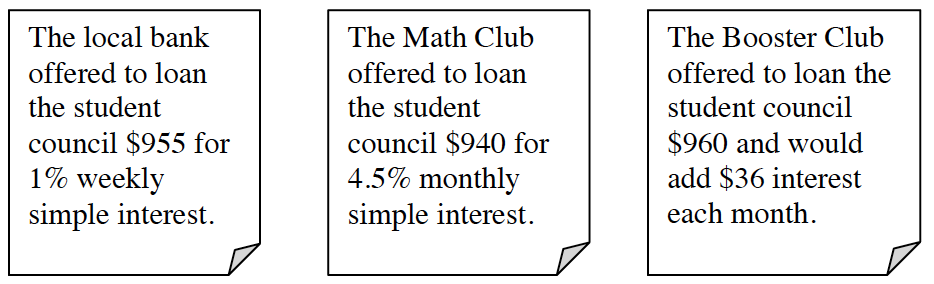a. Which loan would grow most quickly?  That is, which has the most interest added each month?

b. What is the monthly interest rate the Booster Club is offering?  Show how you got your answer.

c. For which loan would the student council owe the most money overall after 3 months?  Justify your answer.

Assignment:

[/expand]

[expand title=”What is the missing number?”]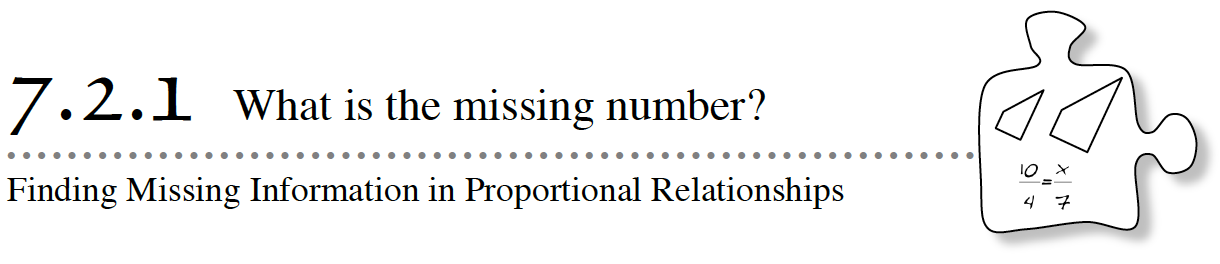7-96. In Chapter 4, Kaci was trying to figure out how many pounds of Havarti cheese she could buy for \$10, knowing that 3 pounds costs \$7.50.  She wrote the equivalent ratios below.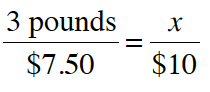Work with your team to brainstorm a list of all of the methods that you can think of that Kaci could use to find the information she is missing.  Be prepared to share your ideas with the rest of the class.

7-97. Graeme knows that he earns \$4.23 every half hour.  He wanted to know how much money he would earn after one full week of work (40 hours).  He wrote the equivalent ratios below and quickly sees a way to find his answer.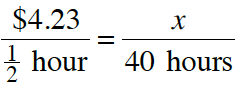a. What do you think Graeme is thinking of doing?

b. Solve Graeme’s equation.

c. Graeme wants to know how long it will take him to earn \$1000.  Write and solve an equation to help him figure it out.

7-98. Shiloh knows that it takes 5 cups of flour to make 2 recipes of cookies.  He has 13 cups of flour left in his cupboard.  He wants to know how many recipes he can make.  He wrote the equivalent ratios below.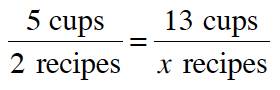This equation looked difficult to solve, so he decided to ask his friend Carolyn for help.  Carolyn said,

“If it takes 5 cups of flour to make 2 recipes, then it takes  2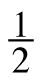cups of flour for each recipe.  This is the multiplier.  Can’t you just use the multiplier to write an equation?”

Carolyn then wrote the equation F = 2R where F stands for cups of flour and R stands for the number of recipes.  As Carolyn wrote the equation below, she said, “Then I would solve this equation to find out the number of recipes.”

13 = 2R

a. Compare Shiloh and Carolyn’s equations.  Are they correct?  Why or why not?

b. Which equation is easiest to solve?  Solve it.

c. Does your solution work in both Shiloh and Carolyn’s equations?

7-99. Find the missing information in each set of equivalent ratios below, using any method.  Be sure that you record your process so that others can see what you did.

1.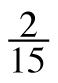=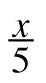2.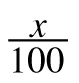=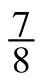3.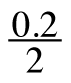=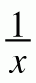4.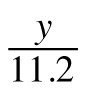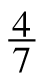Assignment: 7.2.1 Homework

[/expand]

[expand title=”How can I find the missing value?”]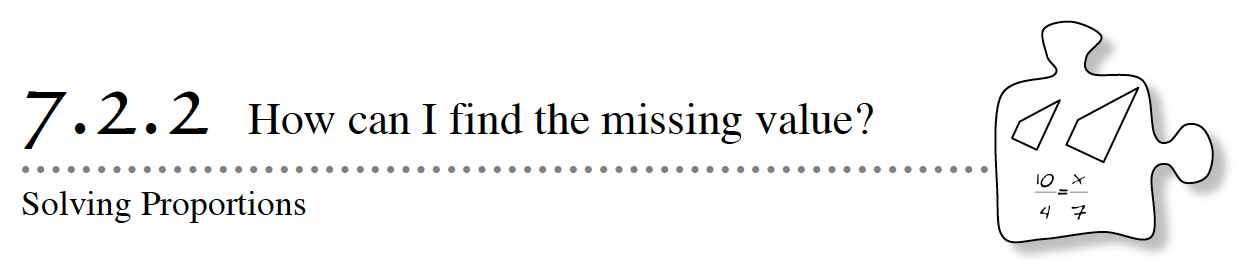You have examined several proportional situations (such as the price of yogurt versus the number of pounds of yogurt) and non-proportional situations (such as the value of a college fund over time).  Today you will continue to develop strategies for solving proportional equations.

7-106. J.R. reduced figure A at right to create similar figure B.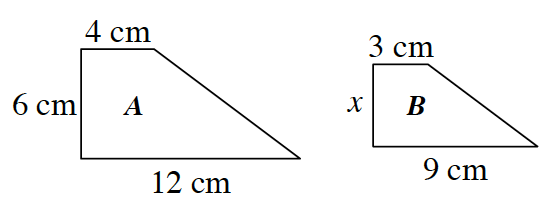a. Write all of the ratios that compare the corresponding sides of figure B to figure A.  What is the relationship between these ratios?  How do you know?

b. One of the relationships between the sides can be written as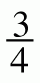=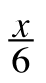.  Find two different ways to find the value of   in this equation.  Is your answer reasonable?  Be ready to share your strategies with the class.

7-108. Solve the problems below.

a. Use Leticia’s method of undoing division to solve this proportion: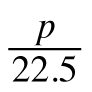=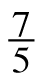.  When you find  p,  replace the value in the original proportion to confirm that the two ratios are equal.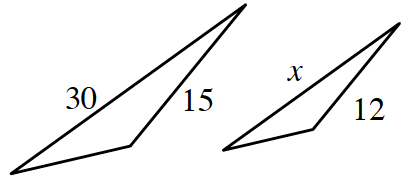b. Write and solve a proportional equation for   for the similar triangles at right.  Then use Avner’s method of undoing both denominators (Fraction Busters) to solve for  x.

c. An apple-juice container has a tiny hole in it, so it is slowly leaking.  If the container leaks three ounces every 19 minutes, how long will it take for the 16 ounces of juice in the container to leak out?  Write a proportional equation and solve.

7-109. For each problem below, decide if the situation is proportional.  If the problem is proportional, say so, and then solve the problem using any strategy you choose.  If the problem is not proportional, explain why not, and then solve the problem.

a. Steve drove 130 miles from Portland to Tacoma in 2 hours.  If he continues to drive at the same speed, how long will it take him to drive 390 miles?

b. At an amusement park, you pay a \$15 entrance fee and then \$4 for each ride you go on.  How much will it cost you to go on seven rides?

c. Armando has collected 39 bottle caps in the past three months.  At this rate, how many bottle caps will he have in five months?

d. The grocery store sells 3 limes for 99 cents.  At this rate, how much will a dozen limes cost?

e. Margarit drove her friends to a movie.  She drove for 30 minutes at 10 miles per hour in heavy traffic, and then she drove for 15 minutes at 40 miles per hour.  How far did she travel in those 45 minutes?

7-110. After Ramona had solved several proportional equations, she noticed a pattern.  “When we eliminated both of the fractions by multiplying by both denominators, we ended up with something that looks like we just multiplied diagonally.”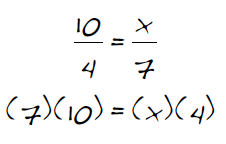a. What does she mean?  For each of the proportions below, apply Ramona’s diagonal multiplying pattern and determine whether the result is a true mathematical equation.  Will her pattern always work?

1.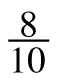=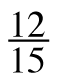2.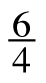=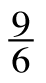3.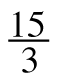=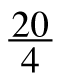b. Use Ramona’s pattern to solve the equation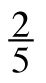=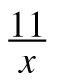for  x.

Assignment: HW 7.2.2

[/expand]